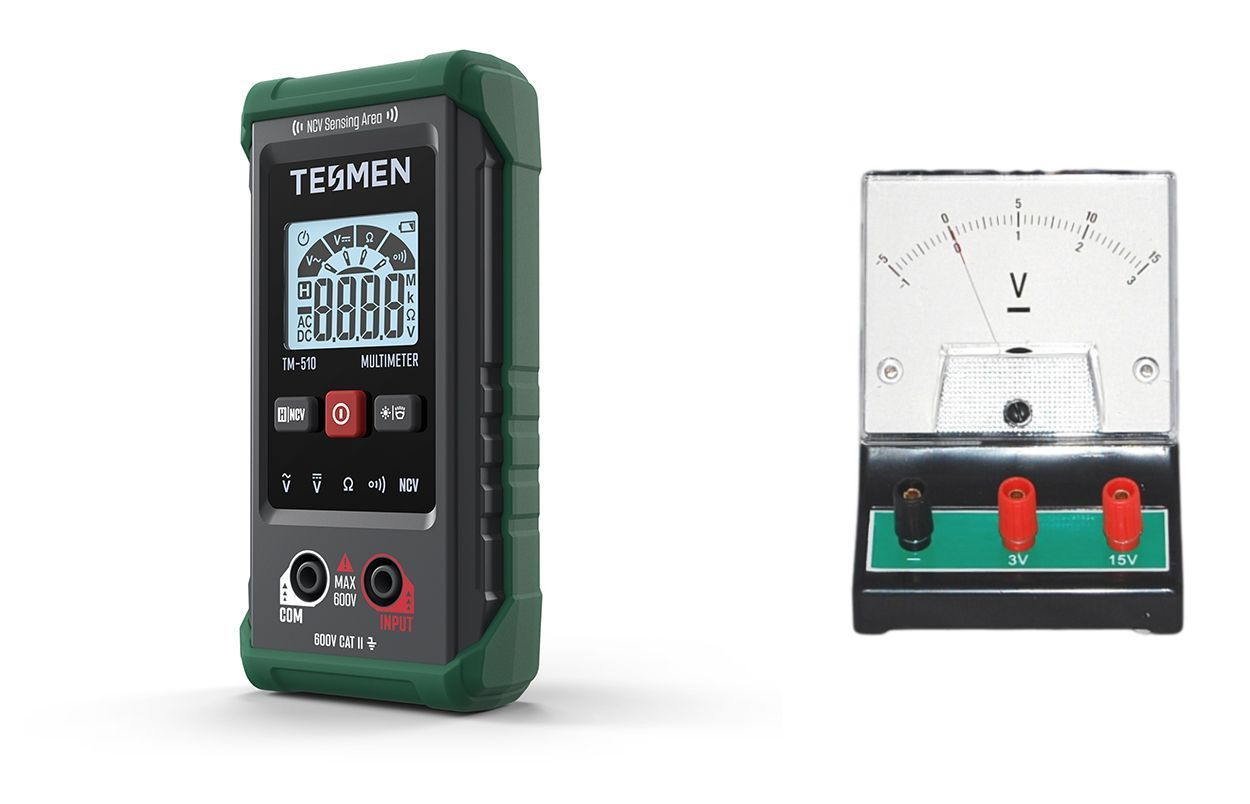# Multimeter VS Voltmeter

Multimeter and voltmeter are two common handheld measuring devices used in electrical work, but they serve different purposes and have distinct features. In this article, we will explore the key differences between these two instruments to help you better understand when and how to use them effectively.

## What is a Multimeter?

A multimeter, short for "multiple meter," is a versatile measuring device that combines various measuring instruments into a single unit. It can measure several electrical quantities, including current, voltage, resistance, and more. Some advanced models of multimeters can even measure properties like transistor gain and diode forward voltage. Electricians often use multimeters to troubleshoot problems in electrical appliances, motors, circuits, and other systems.

A basic multimeter typically includes components such as a DC voltmeter, AC voltmeter, DC ammeter, AC ammeter, and ohmmeter. It can help detect faults in electric circuits and provide measurements in standard units.

## Types of Multimeters

Multimeters can be categorized into two main types based on their design and measurement methods:

1. Analog Multimeter:Analog multimeters utilize a moving coil meter and display measurements using a pointer deflection on an analog scale. They are primarily used for measuring electrical quantities like voltage, current, resistance, frequency, and power.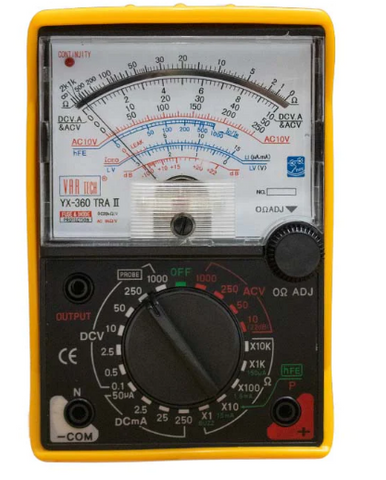1. Digital Multimeter (DMM): A digital multimeter, on the other hand, displays measurement values on a digital screen. It can measure electrical quantities such as voltage, current, and resistance, and it provides accurate and easy-to-read digital readings.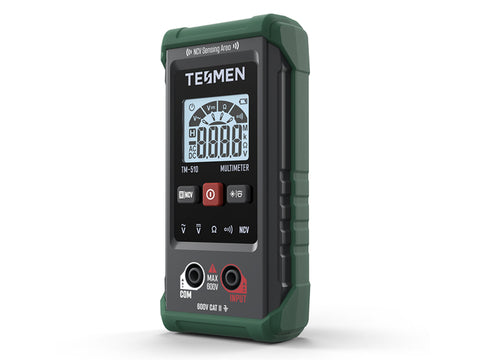## What is a Voltmeter?

A voltmeter is a specialized electrical measuring instrument used exclusively for measuring voltage or electric potential difference between two points in an electrical circuit. It is sometimes simply referred to as a voltage meter. To measure voltage across a device, the voltmeter is connected in parallel with the device so that the same voltage drop occurs across both the device and the voltmeter.

One crucial characteristic of a voltmeter is its very high internal resistance, ensuring that minimal to no current flows through the voltmeter. This high resistance is essential to obtain accurate voltage readings without affecting the circuit being measured.

## Types of Voltmeters

Voltmeters, like multimeters, come in two main types based on their measurement and display methods:

1. Analog Voltmeter:Analog voltmeters provide readings by deflecting a pointer on a scale, which corresponds to the measured voltage.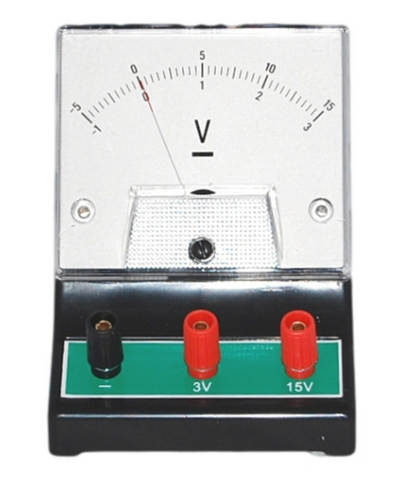1. Digital Voltmeter (DVM):Digital voltmeters display measurement readings on a digital screen, often using a seven-segment display for clarity and precision.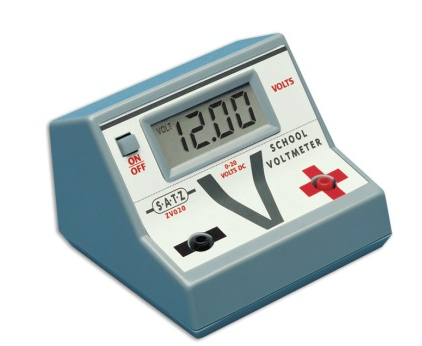## Key Differences between Multimeter and Voltmeter

Let's summarize the key distinctions between multimeters and voltmeters in the following table:

 Parameter Multimeter Voltmeter Definition A versatile device measuring various electrical quantities. Specialized instrument measuring voltage only. Function Combines functions of voltmeter, ammeter, ohmmeter, etc. Solely measures voltage. Circuit Connection Connection varies based on the quantity to be measured. Always connected in parallel to the device. Internal Resistance Variable based on function. High internal resistance to prevent current flow. AC and DC Measurement Measures both AC and DC quantities. Requires separate meters for AC and DC voltage. Construction Complex due to multiple functions. Relatively simple with one circuit. Power Supply Needed Requires a power supply (for digital multimeters). Does not require an external power supply. Size Relatively larger. Smaller. Versatility Versatile, can measure various properties. Limited to measuring voltage. Scale Calibration Calibrated for multiple electrical quantities. Calibrated for voltage measurement. Cost Relatively expensive due to multiple features. Generally cheaper as it measures only voltage. Substitution Can substitute as a voltmeter. Cannot substitute a multimeter. Diode and Transistor Testing Can test diodes and transistors. Cannot test diodes and transistors. Application Used for a wide range of electrical measurements. Primarily used for voltage measurement.

## Conclusion

In conclusion, the difference between a multimeter and a voltmeter is quite straightforward. If you need to measure only voltage in an electric circuit, a voltmeter is the appropriate choice. However, if you require measurements of other properties like current, resistance, capacitance, and more, in addition to voltage, then a multimeter is the versatile instrument to use. Understanding the capabilities and limitations of these tools is essential for efficient electrical troubleshooting and measurement tasks.